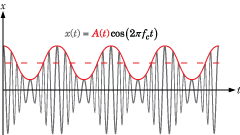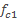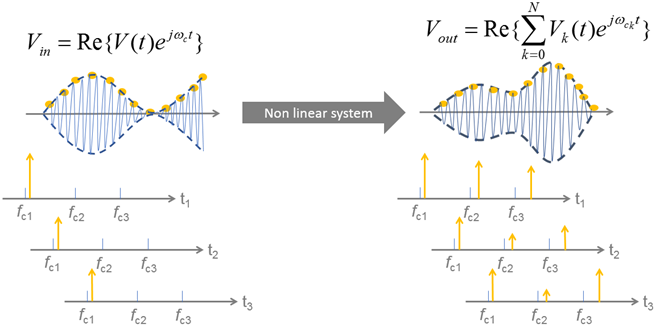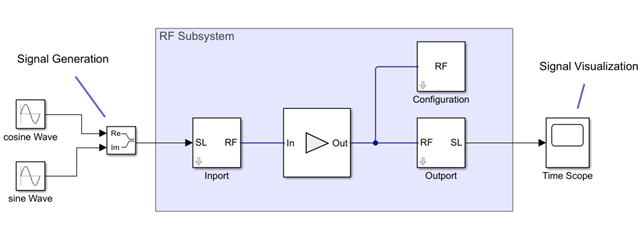## Circuit Envelope Basics

### Introduction

The circuit envelope technique speeds up RF system simulation. In Simulink®, simulating high-frequency signals requires a time step proportional to the highest frequency present in the RF system. However, the modulation frequency or envelope of the RF signals can be several orders of magnitude smaller than the highest frequency. The circuit envelope technique takes advantage of this condition to model RF signals accurately while reducing simulation time.

For example, the following figure shows the benefit of using circuit envelope with a signal x(t):The signal consists of a time-varying modulating signal on a high-frequency carrier or center frequency. In many RF applications, the frequency of the modulating signal, A(t), is smaller than the frequency of the carrier, fc.

RF Blockset™ software handles the carrier cos(2πfct) analytically, so only simulates the modulating signal. RF Blockset uses two methods to handle this simulation, equivalent baseband and circuit envelope. In both methods, the simulation engine takes time steps on the scale of the modulating signal instead of the carrier.

Compared to equivalent baseband simulation, circuit envelope allows including additional non-linear effects beyond in-band spectral regrowth and is suitable for multicarrier simulation.

Using circuit envelope simulation, you can model:

• Even and odd order nonlinear effects, generating in-band, and out-of-band harmonics and spectral regrowth

• Multiport and wideband filtering (frequency selective) effects, like the effects introduced by S-parameters, including impedance mismatches

• In-band and out-of-band interfering and spur signals, including mixing effects

• DC conversion and DC offsets

• Arbitrary local oscillator signals, including phase noise

• Thermal noise generation

• Tunable RF elements controlled by Simulink signals (VGA, switches, RLC, attenuator, phase shifter, etc.)

### Assumptions for Circuit Envelope Simulation

In circuit envelope simulation, you assume that the modulation envelope of the signal varies slowly with respect to the carrier. This type of simulation assumes the signal envelopes to be constant over a single cycle of the carrier (quasi-static assumption). You can assume that a signal envelope is narrowband when it has a frequency at least one order of magnitude smaller than the carrier frequency.

For real passband and wide-band modulated signals, circuit envelope provides correct results. However, the simulation can be slower than traditional time-domain (transient) simulation techniques, like the technique supported by Simulink. If your input is an ultra-wideband signal, or if you deal with many modulated signals filling the simulation spectrum, use time-domain passband simulation.

When considering the quasi-static assumption, you need to consider the effect of the nonlinearities that increase the bandwidth of the signal envelope. Signal envelopes, including in-band spectral regrowth, should be narrowband compared to the carrier frequency. Therefore, circuit envelope is less suitable for simulating hard non-linearities, like nonlinearities resulting from clipping or saturating effects.

In multicarrier signals, overlapping envelopes are not recommended. Overlapping envelopes occur when carrier frequencies are spaced at a distance smaller than the envelope bandwidth. In this case, you can reduce the simulation time step and accommodate the signal information within a single envelope. For more information, see Configuration.

### What Is Circuit Envelope?

Circuit envelope is a time domain (transient) simulation superimposed on a harmonic balance analysis. The analysis is performed at discrete points in time.

Harmonic balance is a frequency-domain method for calculating the steady-state response of non-linear circuits when excited with a finite number of harmonic tones. This analysis solves the system of equations in the frequency domain and is suitable for simulating frequency-defined components such as S-parameters or transmission lines.

Harmonic balance is used by circuit envelope to analyze the system response at every time step. The simulation derives the analysis frequencies from the signal carriers. The harmonic tone coefficients are time varying and processed using transient simulation. This process provides the time-varying envelopes around the harmonic tones.

In the figure below, you see a schematic representation of a circuit envelope simulation. A modulated sinusoidal signal centered aroundis input to a nonlinear system. The output of the system has multiple harmonics, each with a time-varying envelope.In circuit envelope, the time step should be small enough to capture the bandwidth of the envelope and not the maximum frequency (carrier) of the signal. A smaller simulation time step corresponds to a larger simulation (envelope) bandwidth, and thus a slower simulation.

The circuit envelope simulation time step is set in the Configuration block. The simulation time step must be sufficiently small to capture the signal modulation (bandwidth) and the in-band spectral regrowth caused by the system nonlinearity. At the same time, the simulation time step should be as large as possible to increase simulation speed. You can find a tradeoff between accuracy and simulation speed, by using a simulation time step within this range of values

• Use a simulation time step value less than 1/(2*bandwidth) to fulfill Nyquist criterion and correctly sample your signal modulation.

• Use a simulation time step value greater than or equal to 1/(8*bandwidth) to have maximum accuracy at the envelope edges. You can use these ranges of values when simulating S-parameters blocks, filters, and frequency defined components. This time step value also captures the in-band spectral regrowth caused by odd order nonlinearity.

### Time Domain Simulation and Frequency Domain Analysis

Circuit envelope combines time-domain simulation with frequency-domain analysis, there are two edge cases of particular interest.

• If the carrier frequency of all signal sources is 0, then the simulation is reduced to a purely transient (real-passband) simulation with fixed time step. No harmonic balance is performed. This configuration does not speed up the RF system simulation.

• If the simulation stop time is equal to 0, then the simulation is reduced to a purely static nonlinear analysis (harmonic balance) of the system. No time-domain simulation is performed. This configuration is beneficial for the steady-state analysis of the RF system, for example to understand the resulting energy allocation from many signals, or for AC system analysis.

### How Circuit Envelope Simulation Works in RF Blockset

Consider a RF Blockset circuit. You can divide this circuit into three sections: input signal generation, RF subsystem, output signal visualization.#### Input Signal Generation

You can use two kinds of input signal sources:

• RF Blockset signal source

If you are using a Simulink signal source, you need a gateway into the RF subsystem (Inport block). The Simulink input signal represents the modulation of your RF signal. The signal can be complex or real based on the information it carries. To model a constant modulation on a carrier in the Circuit Envelope simulation environment use Continuous Wave block. You can also model noise using current or voltage noise source in RF systems using Noise block.

If the input signal is a vector, each element of the vector represents the envelope signal to be modulated around a certain carrier frequency. You specify the carrier frequencies of the input signal in the Inport block.

#### RF Subsystem

The RF subsystem (highlighted in blue) consists of three main blocks: Inport, Configuration, and Outport. You can include as many Inport or Outport blocks as you need in your RF subsystem.

• `Inport`: The Inport block performs an ideal frequency shift of the input signal around the carrier frequency by implementing a complex frequency multiplication. If your input signal is a vector with each element representing a separate envelope, you can specify separate carrier frequencies for each of them.

You can also specify a zero-carrier frequency corresponding to a real passband signal. In this case, the imaginary part of the input signal is neglected.

• `Configuration`: You use the Configuration block to specify the following:

• The simulation time step, which determines the bandwidth of the envelope simulation. All signal envelopes have the same bandwidth. To avoid resampling of the input signal and aliasing, use the same time step as the signal inputs from Simulink for multiple inport systems. For single inport systems, use slower input signals with interpolation filter checkbox.

• The harmonic order of simulation, which determines the total number of simulation frequencies used to perform the harmonic balance analysis. The auto option gives a conservative choice of harmonic frequencies. The simulation time is directly proportional to the total number of simulation frequencies. If your circuit operates in mildly nonlinear conditions, you can speed up the simulation by reducing the harmonic order.

• Normalization of the carrier power, used to scale the average signal power with respect to its root mean squared value. Use this option to scale the average power of the signal envelope with respect to the root mean square power of its real passband representation.

• The temperature and the seed used for generating thermal noise.

• `Outport`: The Outport block is the return gateway from circuit envelope to the Simulink environment. If you specify a carrier frequency other than zero, the block returns the complex envelope of the signal around the specified carrier. Outport blocks are used to probe modulated signals on the specified carriers for viewing using the Spectrum Analyzer or for further signal processing.

#### Output Signal Visualization

You can use Simulink sinks to visualize the RF signals. To access circuit envelope signals in Simulink, you need an outport block to act as a gateway out of the RF subsystem. The Outport block probes the signal envelopes centered around the specified carrier frequencies.

By using a time scope, you can inspect the time-varying content of the modulated signals without plotting the respective carrier frequency.

By using a spectrum analyzer, you can inspect the spectral content of the modulated signals, which are implicitly centered around the different carrier frequencies.